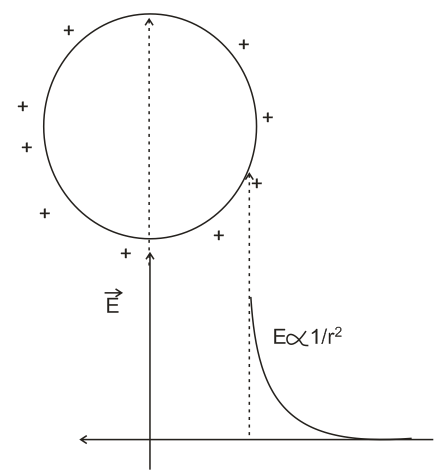# A hollow metal sphere if radius R is uniformly  charged . The electricity field due to the sphere at a distance r from the center :  Option 1) Increases as r increases  for r < R and for r > R  Option 2) zero as r increases for r <  R , decreases as r increases for r > R  Option 3) zero as r increases for r < R , increases as r  > R  Option 4) decreases as r increases for r < R and for r > R

Inside sphere field is zero since there is no change inside , charge get distributed on the surfaceOption 1)

Increases as r increases  for r < R and for r > R

Option 2)

zero as r increases for r <  R , decreases as r increases for r > R

Option 3)

zero as r increases for r < R , increases as r  > R

Option 4)

decreases as r increases for r < R and for r > R

### Preparation Products

##### Knockout NEET May 2021 (One Month)

An exhaustive E-learning program for the complete preparation of NEET..

₹ 14000/- ₹ 6999/-
##### Knockout NEET May 2021

An exhaustive E-learning program for the complete preparation of NEET..

₹ 22999/- ₹ 14999/-
##### Test Series NEET May 2021

Take chapter-wise, subject-wise and Complete syllabus mock tests and get in depth analysis of your test..

₹ 6999/- ₹ 4999/-
##### Rank Booster NEET 2021

This course will help student to be better prepared and study in the right direction for NEET..

₹ 13999/- ₹ 9999/-